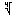# U+221C FOURTH ROOT

U+221C was added to Unicode in version 1.1 (1993). It belongs to the blockMathematical Operators in the Basic Multilingual Plane.

This character is a Math Symbol and is commonly used, that is, in no specific script.

The glyph is not a composition. It has a Neutral East Asian Width. In bidirectional context it acts as Other Neutral and is mirrored. In text U+221C behaves as Alphabetic regarding line breaks. It has type Other for sentence and Other for word breaks. The Grapheme Cluster Break is Any.

The Wikipedia has the following information about this codepoint:

In mathematics, the nth root of a number x, where n is a positive integer, is a number r which, when raised to the power n yields x

where n is the degree of the root. A root of degree 2 is called a square root and a root of degree 3, a cube root. Roots of higher degree are referred by using ordinal numbers, as in fourth root, twentieth root, etc.

For example:

• 2 is a square root of 4, since 22 = 4.
• −2 is also a square root of 4, since (−2)2 = 4.

A real number or complex number has n roots of degree n. While the roots of 0 are not distinct (all equaling 0), the n nth roots of any other real or complex number are all distinct. If n is even and x is real and positive, one of its nth roots is positive, one is negative, and the rest are complex but not real; if n is even and x is real and negative, none of the nth roots is real. If n is odd and x is real, one nth root is real and has the same sign as x , while the other roots are not real. Finally, if x is not real, then none of its nth roots is real.

Roots are usually written using the radical symbol or radix or , with or denoting the square root, denoting the cube root, denoting the fourth root, and so on. In the expression , n is called the index, is the radical sign or radix, and x is called the radicand. Since the radical symbol denotes a function, when a number is presented under the radical symbol it must return only one result, so a non-negative real root, called the principal nth root, is preferred rather than others; if the only real root is negative, as for the cube root of –8, again the real root is considered the principal root. An unresolved root, especially one using the radical symbol, is often referred to as a surd or a radical. Any expression containing a radical, whether it is a square root, a cube root, or a higher root, is called a radical expression, and if it contains no transcendental functions or transcendental numbers it is called an algebraic expression.

In calculus, roots are treated as special cases of exponentiation, where the exponent is a fraction:

Roots are particularly important in the theory of infinite series; the root test determines the radius of convergence of a power series. Nth roots can also be defined for complex numbers, and the complex roots of 1 (the roots of unity) play an important role in higher mathematics. Galois theory can be used to determine which algebraic numbers can be expressed using roots, and to prove the Abel-Ruffini theorem, which states that a general polynomial equation of degree five or higher cannot be solved using roots alone; this result is also known as "the insolubility of the quintic".

## Representations

System Representation
8732
UTF-8 E2 88 9C
UTF-16 22 1C
UTF-32 00 00 22 1C
URL-Quoted %E2%88%9C
HTML-Escape &#x221C;
Wrong windows-1252 Mojibake â

## Complete Record

Property Value
Age (age) 1.1
Unicode Name (na) FOURTH ROOT
Unicode 1 Name (na1)
Block (blk) Math_Operators
General Category (gc) Math Symbol
Script (sc) Common
Bidirectional Category (bc) Other Neutral
Combining Class (ccc) Not Reordered
Decomposition Type (dt) None
Decomposition Mapping (dm)Lowercase (Lower)
Simple Lowercase Mapping (slc)Lowercase Mapping (lc)Uppercase (Upper)
Simple Uppercase Mapping (suc)Uppercase Mapping (uc)Simple Titlecase Mapping (stc)Titlecase Mapping (tc)Case Folding (cf)ASCII Hex Digit (AHex)
Alphabetic (Alpha)
Bidi Control (Bidi_C)
Bidi Mirrored (Bidi_M)
Bidi Paired Bracket (bpb)Bidi Paired Bracket Type (bpt) None
Cased (Cased)
Composition Exclusion (CE)
Case Ignorable (CI)
Full Composition Exclusion (Comp_Ex)
Changes When Casefolded (CWCF)
Changes When Casemapped (CWCM)
Changes When NFKC Casefolded (CWKCF)
Changes When Lowercased (CWL)
Changes When Titlecased (CWT)
Changes When Uppercased (CWU)
Dash (Dash)
Deprecated (Dep)
Default Ignorable Code Point (DI)
Diacritic (Dia)
East Asian Width (ea) Neutral
Extender (Ext)
FC NFKC Closure (FC_NFKC)Grapheme Cluster Break (GCB) Any
Grapheme Base (Gr_Base)
Grapheme Extend (Gr_Ext)
Hex Digit (Hex)
Hangul Syllable Type (hst) Not Applicable
Hyphen (Hyphen)
ID Continue (IDC)
Ideographic (Ideo)
ID Start (IDS)
IDS Binary Operator (IDSB)
IDS Trinary Operator and (IDST)
InMC (InMC)
Indic Positional Category (InPC) NA
Indic Syllabic Category (InSC) Other
ISO 10646 Comment (isc)
Joining Group (jg) No_Joining_Group
Join Control (Join_C)
Jamo Short Name (JSN)
Joining Type (jt) Non Joining
Line Break (lb) Alphabetic
Logical Order Exception (LOE)
Math (Math)
Noncharacter Code Point (NChar)
NFC Quick Check (NFC_QC) Yes
NFD Quick Check (NFD_QC) Yes
NFKC Casefold (NFKC_CF)NFKC Quick Check (NFKC_QC) Yes
NFKD Quick Check (NFKD_QC) Yes
Numeric Type (nt) None
Numeric Value (nv) NaN
Other Alphabetic (OAlpha)
Other Default Ignorable Code Point (ODI)
Other Grapheme Extend (OGr_Ext)
Other ID Continue (OIDC)
Other ID Start (OIDS)
Other Lowercase (OLower)
Other Math (OMath)
Other Uppercase (OUpper)
Pattern Syntax (Pat_Syn)
Pattern White Space (Pat_WS)
Quotation Mark (QMark)
Radical (Radical)
Sentence Break (SB) Other
Simple Case Folding (scf)Script Extension (scx) Common
Soft Dotted (SD)
STerm (STerm)
Terminal Punctuation (Term)
Unified Ideograph (UIdeo)
Variation Selector (VS)
Word Break (WB) Other
White Space (WSpace)
XID Continue (XIDC)
XID Start (XIDS)
Expands On NFC (XO_NFC)
Expands On NFD (XO_NFD)
Expands On NFKC (XO_NFKC)
Expands On NFKD (XO_NFKD)